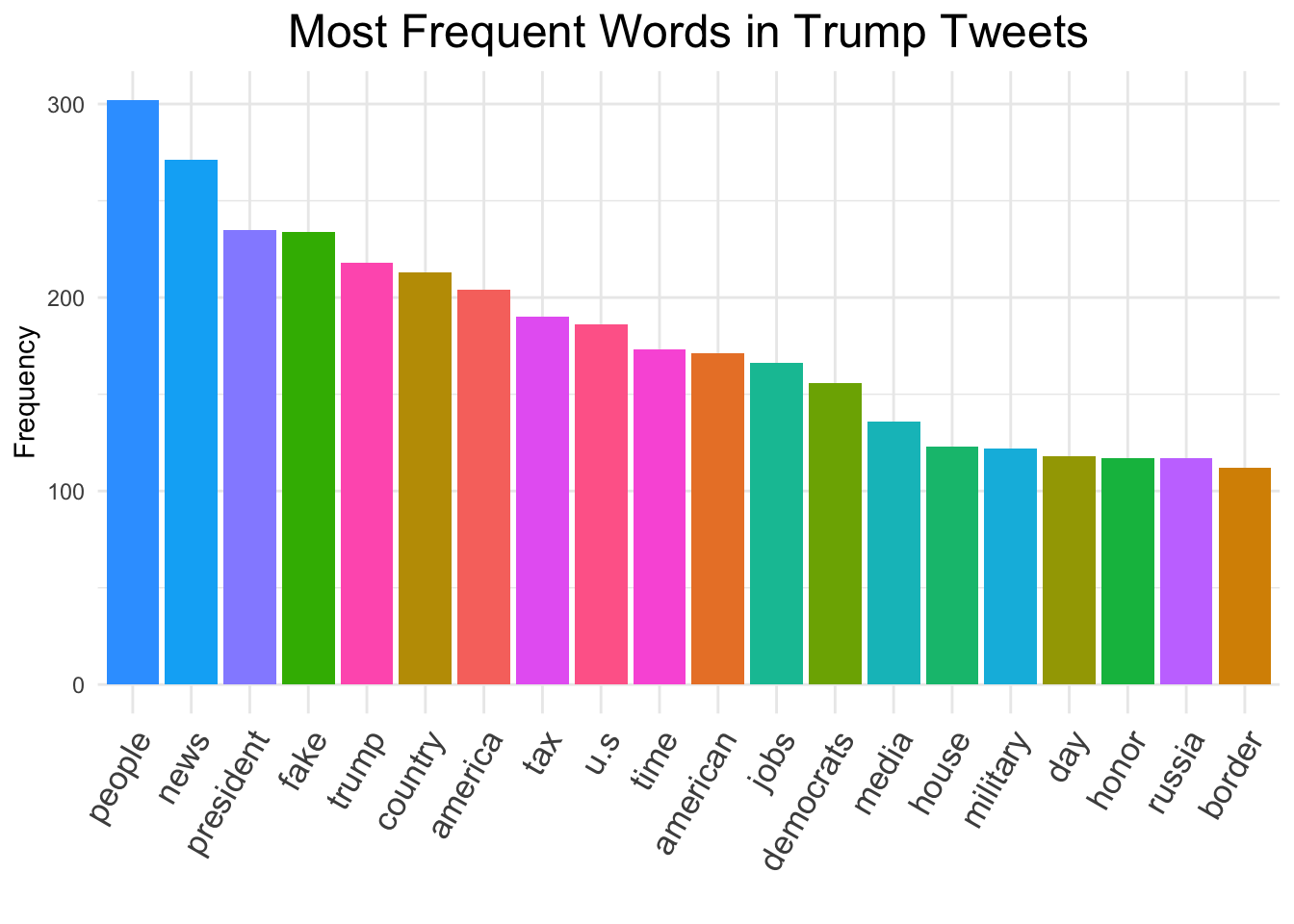Chris Bail, PhD
Duke University
www.chrisbail.net
github.com/cbail

## Introduction

Among the most basic forms of quantitative text analysis are word-counting techniques and dictionary-based methods. This tutorial will cover both of these topics, as well as sentiment analysis, which is a form of dictionary-based text analysis. This tutorial assumes basic knowledge about R and other skills described in previous tutorials at the link above.

## Word Counting

In the early days of quantitative text analysis, word-frequency counting in texts was a common mode of analysis. In this section, we’ll learn a few basic techniques for counting word frequencies and visualizing them. We’re going to work within the `tidytext` framework, so if you need a refresher on that, see my previous tutorial entitled “Basic Text Analysis in R.”

Let’s begin by loading the Trump tweets we extracted in a previous tutorial and transform them into `tidytext` format:

``````load(url("https://cbail.github.io/Trump_Tweets.Rdata"))
library(tidytext)
library(dplyr)
tidy_trump_tweets<- trumptweets %>%
select(created_at,text) %>%
unnest_tokens("word", text)``````

Next, let’s count the top words after removing stop words (frequent words such as “the”, and “and”) as well as other unmeaningful words (e.g. https):

``````data("stop_words")

top_words<-
tidy_trump_tweets %>%
anti_join(stop_words) %>%
filter(!(word=="https"|
word=="rt"|
word=="t.co"|
word=="amp")) %>%
count(word) %>%
arrange(desc(n))``````

Now let’s make a graph of the top 20 words

``library(ggplot2)``
``## Warning: package 'ggplot2' was built under R version 3.5.2``
``````top_words %>%
slice(1:20) %>%
ggplot(aes(x=reorder(word, -n), y=n, fill=word))+
geom_bar(stat="identity")+
theme_minimal()+
theme(axis.text.x =
element_text(angle = 60, hjust = 1, size=13))+
theme(plot.title =
element_text(hjust = 0.5, size=18))+
ylab("Frequency")+
xlab("")+
ggtitle("Most Frequent Words in Trump Tweets")+
guides(fill=FALSE)``````## tf-idf

Though we have already removed very common “stop words” from our analysis, it is common practice in quantitative text analysis to identify unusual words that might set one document apart from the others (this will become particularly important when we get to more advanced forms of pattern recognition in text later on). As the figure below shows, the metric most commonly used to identify this type of words is “Term Frequency Inverse Document Frequency” (tf-idf).